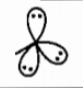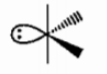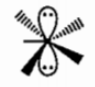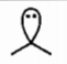Select the incorrect match:

Statement                               Shape                                    Example

(a) Bond pair has > 75%HF

p-character

(b) Reduction in axial bond${\mathrm{SF}}_{4}$

is more than that of in

equatorial bond angle

(c) Two axial d-orbitals and${\mathrm{XeF}}_{5}^{-}$

one non-axial d-orbitals are

used in hybridization

(d) Two p-orbitals are used${\mathrm{SnCl}}_{2}$

in hybridization